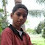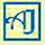# CBSE Xth Science - Chemistry | Chapter 2 Acids, Bases and Salts | NCERT Solutions for In-Text Questions

## Chapter 2, Acids, Bases and Salts

### Solutions of NCERT Science Textbook Chapter In-Text Questions

(Page 18)
QUESTION.1: You have been provided with three test tubes. One of them contains distilled water and the other two contain an acidic and basic solution, respectively. If you are given only red litmus paper, how will you identify the contents of each test tube?
Solution: First of all, we put one strip of red litmus paper in each test tube. In one of the test tubes, the litmus paper will turn blue which indicates the solution in that test tube is basic. Out of the remaining two test tubes any one can be acid or neutral (distilled water). Now a drop of basic solution is mixed with a drop of each of the remaining two solutions separately. Then we check the nature of the drops of the mixture again with red litmus. There can be two results:
1. If the colour of red litmus turns blue, then the second solution is neutral (distilled water), and
2. If there is no change in colour and the litmus remains red, then the second solution is acidic. This is because acidic and basic solutions neutralize each other.
Thus, we can identify the contents of each test tube.
(Page 22)
QUESTION.1: Why should curd and sour substances not be kept in brass and copper vessels?
Solution: Curd and sour substances contain acids. Therefore, if we keep such food substances in brass and copper vessels, they will react with the metal to form poisonous metal compounds and liberate hydrogen gas thereby causing food poisoning and corrosion of the vessels.
QUESTION.2: Which gas is usually liberated when an acid reacts with a metal? Illustrate with an example. How will you test for the presence of this gas?
Solution: Usually, hydrogen gas is liberated when an acid reacts with a metal. For example, let us take the reaction between zinc and sulphuric acid, as per the apparatus set-up shown in the adjacent figure.
Source: NCERT Science Textbook
A few pieces of zinc granules are taken in a test tube and about 5 mL of dilute H2SO4 is added. The gas produced is passed into a soap solution. We find that bubbles of soap solutions are formed. When a burning candle is brought near the soap bubbles, it burns with a pop sound which confirms the presence of hydrogen gas.
Zinc granules react with dilute sulphuric acid to for zinc sulphate and hydrogen gas according to the chemical; equation:
Zn + H2SO4 ---> ZnSO4 + H2
QUESTION.3: A metal compound reacts with dilute Hydrochloric Acid to produce effervescence. The gas evolved extinguishes a burning candle. Write a balanced chemical equation for the reaction if one of the compounds formed is Calcium Chloride.
Solution: CaCO3 + 2HCl  ---> CaCl2 + H2O + CO2
(Page 25)
QUESTION.1: Why do HCl, HNO3, etc. show acidic characters in aqueous solutions while solutions of compounds like alcohol and glucose do not show acidic character?
Solution: HCl and HNO3 etc. dissociate in presence of water to form hydrogen ions (H+). These hydrogen ions combine with H2O to form hydronium ions (H3O+). The reaction can be given as follows:
HCl ---> H+ + Cl
H+ + H2O ---> H3O+
Because of this property HCl and HNO3 show acidic character in aqueous solutions. On the other hand, alcohol and glucose can not dissociate in water to form hydrogen ions. Hence, they do not show acidic character.
QUESTION.2: Why does an aqueous solution of acid conduct electricity?
Solution: When dissolved in water, acids dissociate to form ions e.g.,
HCl + H2O ---> Cl + H3O+
These ions are responsible for electrical conductivity.
QUESTION.3: Why does dry HCl gas not change the colour of the dry litmus paper?
Solution: We know that the colour of the litmus is changed by H+ ions of an acid. Dry HCl does not dissociate to give H+ ions. It is only in the aqueous that an acid dissociate to give ions. Since in this case, neither HCL is in aqueous form nor is the litmus paper wet, so the colour of litmus paper does not change.
QUESTION.4: While diluting an acid, why is it recommended that the acid should be added to water and not water to the acid?
Solution: The process of dissolving an acid or a base in water is a highly exothermic one. Care must be taken while mixing concentrated HNO3 acid or H2SO4 with water. The acid must always be added slowly to water with constant stirring. If water is added to a concentrated acid, the heat generated may cause the mixture to splash out and cause burns. The glass container may also break due to excessive local heating.
QUESTION.5: How is the concentration of hydronium ions (H3O+) affected when a solution of an acid is diluted?
Solution: Concentration of hydronium ions (H3O+) decreases. As a result the acid is diluted.
QUESTION.6: How is the concentration of hydroxide ions (OH) affected when excess base is dissolved in a solution of sodium hydroxide?
Solution: Concentration of hydroxide ions (OH) increases.
(Page 28)
QUESTION.1: You have two solutions, A and B. The pH of solution A is 6 and pH of solution B is 8. Which solution has more hydrogen ion concentration? Which of this is acidic and which one is basic?
Solution: Solution A has more H+ ion concentration. A pH value of less than 7 indicates an acidic nature while greater than 7 indicates basic nature of a solution. So, solution A is acidic and solution B is basic.
QUESTION.2: What effect does the concentration of H+ (aq) ions have on the nature of the solution?
Solution: As the concentration of H+ ions increases the solution becomes more acidic while a decrease of H+ ion causes an increase in the basicity of the solution.
QUESTION.3: Do basic solutions also have H+ (aq) ions? If yes, then why are these basic?
Solution: Yes, basic solutions also have H+ ions. However, their concentration is less as compared to the OH ions that makes the solution basic.
QUESTION.4: Under what soil condition do you think a farmer would treat the soil of his fields with quick lime (Calcium Oxide) or slaked lime (Calcium Hydroxide) or chalk (Calcium Carbonate)?
Solution: If the farmer finds his soil turned acidic, then to increase the Basicity of soil, he should use the above materials to neutralize it.
(Page 33)
QUESTION.1: What is the common name of the compound CaOCl2?
Solution: Bleaching powder.
QUESTION.2: Name the substance which on treatment with chlorine yields bleaching powder.
Solution: Dry slaked lime [Ca(OH)2].
QUESTION.3: Name the sodium compound which is used for softening hard water.
Solution: Washing soda i.e. sodium carbonate (Na2CO3.10H2O).
QUESTION.4: What will happen if a solution of sodium hydrocarbonate is heated? Give the equation of the reaction involved.
Solution: The following reaction takes place when it is heated:
QUESTION.5: Write an equation to show the reaction between Plaster of Paris (POP) and water.
Solution: The chemical equation for the reaction of Plaster of Paris and water can be represented as follows:
CaSO4.½H2O + 1½H2O ---> CaSO4.2H2O

Further Study on Chapter 2, Acids, Bases and Salts
• Xth CBSE Chemistry | Chapter 2, Acids, Bases and Salts | NCERT Science Textbook Exercise Solution [Read]
• Acids, Bases and Salts | Class 10, NCERT (CBSE) Science - Chemistry | Sample Questions and Extrascore Notes [Read]
• Acids, Bases and Salts | Xth NCERT (CBSE) Science - Multiple Choice Questions (MCQ) [Read]

1.anybody pls help me!!!!

2.We understand the importance of these answers. But due to some unavoidable reasons it is taking time to publish the answers. We are updating them asper the latest CCE pattern and we assure you will find them here within next ten days. Thanks for bearing with us.

3.4.5.